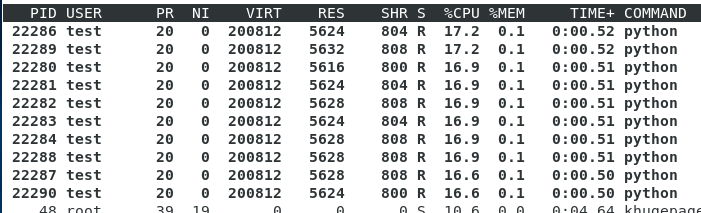2020-05-13 16:47

# 多进程在运行的时候只有一个子进程会运行，怎么解决？

``````if __name__ == '__main__':
print('Started at ', datetime.now())

processes = []
for i in range(0,10):
t = multiprocessing.Process(target = mainFunction, args = (i,))
processes.append(t)
for t in processes:
t.start()
t.join()

print('Finished at ', datetime.now())

``````

• 点赞
• 写回答
• 关注问题
• 收藏
• 邀请回答

#### 1条回答默认 最新

•bobhuang 2020-05-13 18:14
已采纳

这段代码，每次循环都是start一个线程，并等待结束后再开始下一个线程。

``````    for t in processes:
t.start()
t.join()
``````

改为：

``````    for t in processes:
t.start()
for t in processes:
t.join()
``````

完整的测试代码:

``````import time
import multiprocessing
from datetime import datetime

def mainFunction(idx,running):
while(running.value):
pass

if __name__ == '__main__':
running = multiprocessing.Value('i',1)
print('Started at ', datetime.now())
processes = []
for i in range(0,10):
t = multiprocessing.Process(target = mainFunction, args = (i,running,))
processes.append(t)
for t in processes:
t.start()

time.sleep(10)
running.value = 0
``````

运行结果:

``````('Started at ', datetime.datetime(2020, 5, 14, 11, 9, 47, 862220))